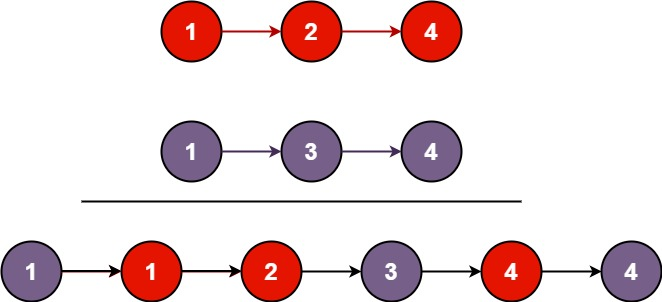21. Merge Two Sorted Lists
Easy
17.7K
1.6K

You are given the heads of two sorted linked lists `list1` and `list2`.

Merge the two lists in a one sorted list. The list should be made by splicing together the nodes of the first two lists.

Example 1:```Input: list1 = [1,2,4], list2 = [1,3,4]
Output: [1,1,2,3,4,4]
```

Example 2:

```Input: list1 = [], list2 = []
Output: []
```

Example 3:

```Input: list1 = [], list2 = 
Output: 
```

Constraints:

• The number of nodes in both lists is in the range `[0, 50]`.
• `-100 <= Node.val <= 100`
• Both `list1` and `list2` are sorted in non-decreasing order.
Accepted
3.1M
Submissions
5M
Acceptance Rate
62.5%

Seen this question in a real interview before?
1/4
Yes
No

Discussion (0)

Related Topics